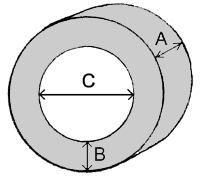tyre size, coding, width, ration, diameter, information.

# Tyre size coding information explained.

To automatically decode tyre size codes, use this Tyre Size Decoder app.

A tyre has a code such as:
"185/60R/15H"
A/B/CA = Width of tyre (in millimetres) In this example it shows that the tyre is 185mm wide. B = Tyre profile as a percentage of width (A). In this example the ratio is 60% of 185mm. This works out as 111mm. C = Diameter of inner rim (in inches). In this example it shows that the rim is 15" in diameter.   From this you can also work out your rolling circumference. e.g. (2 × B) + C = diameter = d therefore (2 × 111) + (15" × 25.4) = 222 + 381 = 603mm circumference = π × d = 3.142 × 603 = 1895mm
Website Design by K300